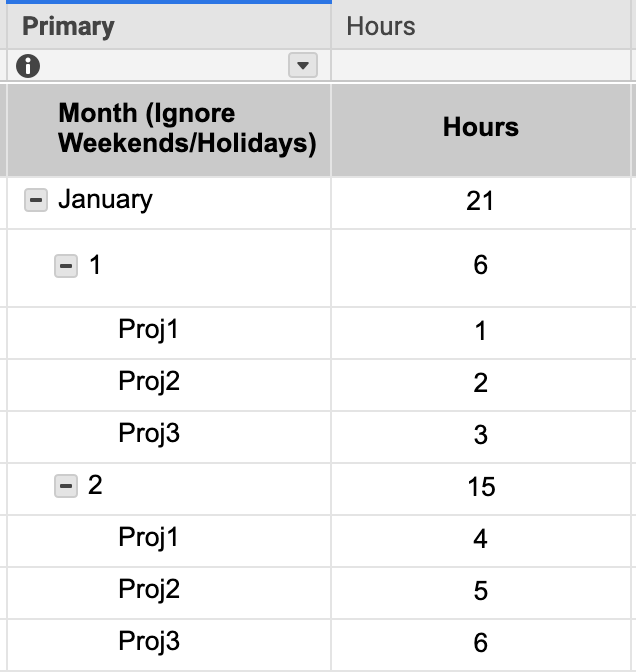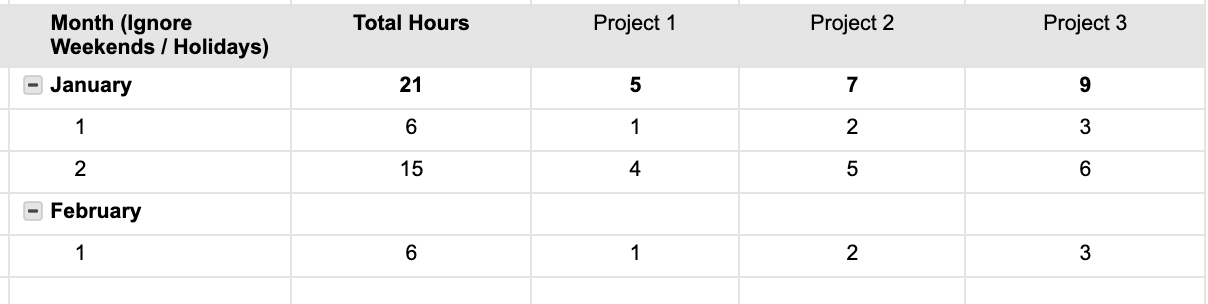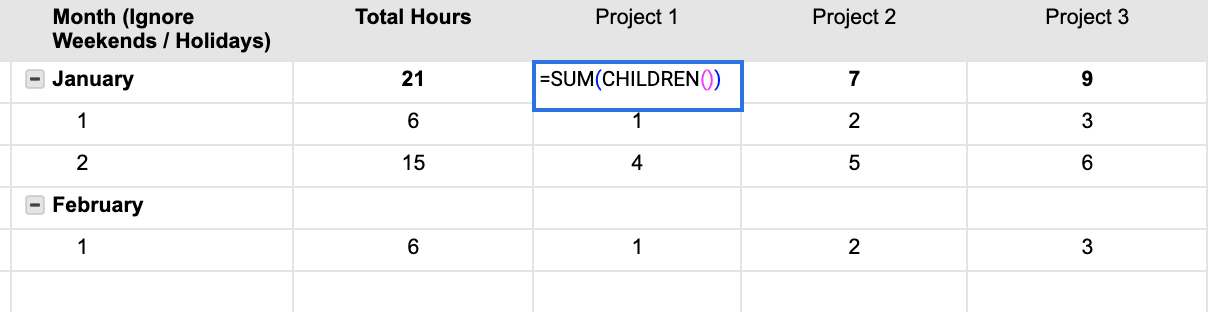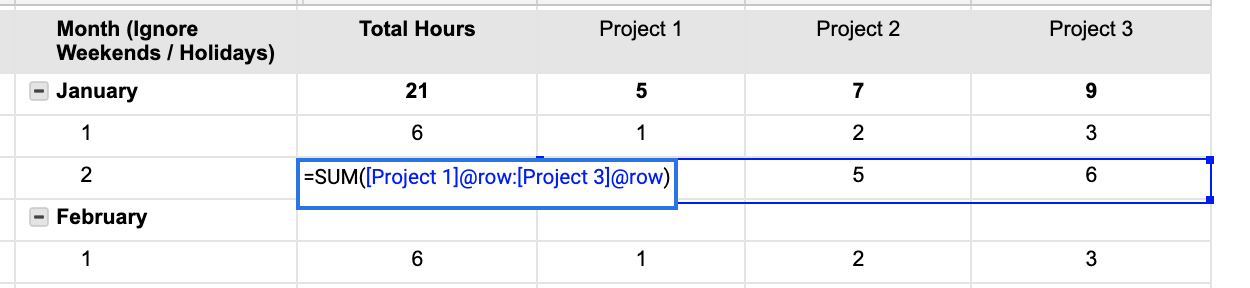# sum hours from a column, if grandchild row in another column has specific text

So I've been tasked with creating a timesheet for our consultant agency by quarter, so each sheet will cover three months, why it has to be in Smartsheet I'm not sure.

Context

Each day, employees have three types of projects they can be working on: "Proj1", "Proj2", "Proj3".

I need a formula that will add up all the project hours for the month of January.

In the dummy data from the image, you can see there were 21 hours total worked in January. I need to figure out how many of them were on Proj1 (should be 5).

Formula

However I can't seem to find a formula that works for this.

I came close with this formula: =SUMIF(Primary:Primary, "Proj1", Hours:Hours)

It works until hours from February are added, then they end up being included. I need to find a way to search the grandchildren rows of the January parent, where the project types are listed for say, "Proj1" and add those hours.

I'm thinking something like: =SUMIF(CHILDREN(Primary2), FIND("gUP"), Hours:Hours) but I know I'm getting it wrong.Tags:

• Would it be possible to adjust the organization of the sheet? For example, if there will only ever be the three projects worked on, could these be column names instead of repeated "grandchildren" rows, which would make it quite simple to calculate the correct data.

In this instance, the parent row with January could rollup a total for each project with the SUM(CHILDREN() function. You could have a "Total Hours" column with the three projects added together for that specific day, too.

This would have a formula to add up the three project hours for that day, such as

=SUM([Project 1]@row:[Project 3]@row)

Let me know if this would work for you.

Cheers,

Genevieve• You could also add in a helper column with a formula such as

=IF(COUNT(CHILDREN(Primary@row) > 0, PARENT(Primary@row), PARENT())

.

In the rows where you have 1, 2, etc, it will pull "January" from the Primary column (or whatever month the primary is). In the rows where you have the Projects (no child rows), it will pull from the parent row in this helper column.

.

This is basically adding the month to every row.

.

Then you can use a SUMIFS such as

=SUMIFS(Hours:Hours, Primary:Primary, @cell = "Proj1", Helper:Helper, @cell = "January")

• Thank you for taking the time to respond! This looks like it would be much more simple, I will have to run it by my project lead to see if it would be acceptable. Thanks for the response!

• Thanks Paul, I'll give this a shot! I appreciate you taking time out of your day to respond.

However when I try to use your formula:

=IF(COUNT(CHILDREN(Primary@row) > 0, PARENT(Primary@row), PARENT()))

I get an error that says "incorrect argument set"

I thought it may have been missing a parenthesis:

=IF(COUNT(CHILDREN(Primary@row) > 0, PARENT(Primary@row)), PARENT())

Which gets rid of the incorrect argument error, but outputs nothing to the cell. I ended up just copying the month name into the helper column manually and everything seems to work as intended, so thank you for your help!

• That's my fault. I missed a closing parenthesis.

Remove 1 from the end of the formula and add it to after the first CHILDREN() function.

=IF(COUNT(CHILDREN()) > 0, PARENT(Primary@row), PARENT())

## Help Article Resources

Want to practice working with formulas directly in Smartsheet?

Check out the Formula Handbook template!